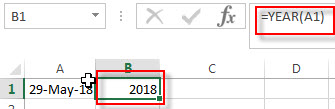# Excel YEAR Function

This post will guide you how to use Excel YEAR function with syntax and examples in Microsoft excel.

### Description

The Excel YEAR function returns a four-digit year from a given date value, the year is returned as an integer ranging from 1900 to 9999. So you can use the YEAR function to get the year component of a date. You can also use this function to extract the year portion from an Excel date and feed the returned value into another formula.

The YEAR function is a build-in function in Microsoft Excel and it is categorized as a DATE and TIME Function.

The YEAR function is available in Excel 2016, Excel 2013, Excel 2010, Excel 2007, Excel 2003, Excel XP, Excel 2000, Excel 2011 for Mac.

### Syntax

The syntax of the YEAR function is as below:

`=YEAR (serial_number)`

Where the YEAR function arguments is:

• Serial_number -This is a required argument. A valid date value that contains the year component you want to extract. Dates should be entered by using the DATE function, or as results of other formulas or functions. For example, use DATE(2019,3,15) for the 15th day of March, 2019.

Note:

• A serial date is how the Microsoft Excel stores dates and it represents the number of days since 1900-01-01, so the January 1, 1900 date is serial number 1 by default.
• If the serial_number is not a valid Excel date, it will return #VALUE! Error.

### Examples

The below examples will show you how to use Excel YEAR Function to return an integer number that representing the year component of a given Excel date

1# get year of the date in Cell A1 , enter the following formula in Cell B1.

=YEAR(A1)### Related Functions

• Excel WEEKDAY function
The Excel WEEKDAY function returns a integer value representing the day fo the week for a given Excel date and the value is range from 1 to 7.The syntax of the WEEKDAY function is as below:=WEEKDAY (serial_number,[return_type])…
• Excel DAY function
The Excel DAY function returns a day of a date (from 1 to 31).The DAY function is a build-in function in Microsoft Excel and it is categorized as a DATE and TIME Function.The syntax of the DAY function is as below:= DAY (date_value)…
• Excel DATEVALUE Function
The Excel DATEVALUE function returns the serial number of date. And it can be used to convert a date represented as text format into a serial number that recognizes as a date format.The syntax of the DATEVALUE function is as below:=DATEVALUE(date_text)…
• Excel MONTH function
The Excel MONTH function returns the month of a date represented by a serial number. And the month is an integer number from 1 to 12. The syntax of the MONTH function is as below:=MONTH (serial_number)…